I.5.8

8/19/2021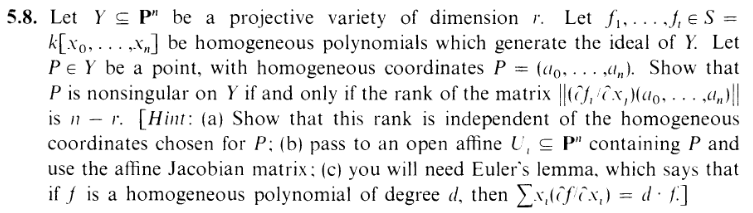p3nor in the B—˙—TT. The formation in place of the "U" is meant to represent duality: One man will see it as that moment in Breakout when your ball goes past a gap in the bricks–that orgasmic moment where you know your ball is about to bounce off the ceiling and wipe out a generous amount off the upper layers on your behalf, and all you have to do is kick your feet up on the paddle and watch the glorious destruction. The other man will realize that the ball is positioned equidistant between the bricks–thus it actually just went straight up through the gap... and it's going to just bounce off the ceiling and come right back down through the gap.

Yes, duality. The glass half full, vs. the glass half empty. Yang vs. Yin. The proposer vs. the contrarian. The Good vs. the Bad. Heads vs. tails. *Flips coin, and catches it between my hands* ...And then there's me. Who am I, you ask? Well, think of me as The Third Man. The Other. The Missing Link. The Forgotten One. The Dark Friend...........................

Shocked? Traumatized? I know. I'm sorry for hiding it from you, but I had no other choice. I had to. Not just for my safety... but for yours. *Grabs your cheeks and brings your face close to mine* I'm sorry.... I just didn't want you to be in danger.... I love you... I love you. *Passionately kisses you on the lips*........ I have to go now. "Wait, not yet!" ........ I have to. It's my duty. "Well.... you better come back, or I'll be really mad!" Heh... Who do you think I am? *Jumps into the darkness*

Okay, the joke is that I tried to type a bar, underscore, and another bar to 1337 a U, but Latex did that weird dash dot dash formation instead.

"BOOOOOOOO, YOU'RE SO SLOW AT MAKING POSTS" Yes, yes, I know. I have not yet reaccustomed myself to my trademark daily posting rate, which... has never actually occurred on this blog...

By the way, some of these exercises were done long back enough that I'm forgetting whether I actually finished them. I almost skipped writing this post because my notes made it look like I gave up. BUT I DIDN'T. Check it out.Let's follow Harty's Hint: The Trilogy. First, we need to show that the choice of homog coords doesn't matter for the rank. Let's denote

 di = deg fi

EVERYONE STOP. I DROPPED MY PROOF.

I just realized that, in my notes, I mistakenly assumed that the partial derivatives would be homogenous. But that's obviously not the case (e.g. differentiate x02 + x1x2 with respect to any variable). Sooooo my argument for (a) breaks down.

Errrr.... Okay... let's just assume (a) for now. RANK IS INDEPENDENT OF CHOICE OF HOMOGENOUS COORDINATES. Fine. Let's just get to the main part. We'll start with the "only if" condition.

###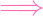Assume P is nonsingular on Y , and assume without loss of generality that it's in the open affine U0 = {x00}, so we can write P = (1,a1,,an) (and thanks to (a), the rank of the matrix will hold for this choice of coordinates).

Let's denote

 gi(x1,…,xn) = fi(1,x1,…,xn)

The gi determine Y U0 (I'll call this set Y 0). So we have two Jacobians in play. One for Y :

 J =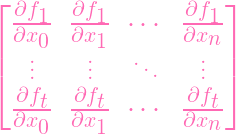(1)

And the other for Y 0:

 J0 =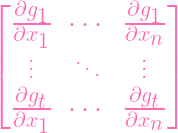(2)

And these matrices are obviously related. For the "only if" () we need to show that rkJ = n - r.

Well, since dim X = dim X, we have that dim Y 0 = dim Y = r. And since Y 0 is affine, it's well known to us that its Jacobian J0 has rank n-r. So really, our problem reduces to rkJ0 = n - rrkJ = n - r. But how is J related to J0?

Recall we set P = (1,a1,,an) Y . Let's get the corresponding point for Y 0 : Q = (a1,,an) (we know it's in Y 0 because it satisfies the gi).

Now, reader, CONVINCE YOURSELF OF THIS: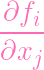(P) =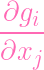(Q) (when j≠0)

Indeed, when you differentiate with respect to a variable that isn't x0, those terms stay constant. So it doesn't matter if you plug in 1 after differentiating to obtain the left hand side, or before differentiating to obtain the right hand side.

Hence we have

 J(P) =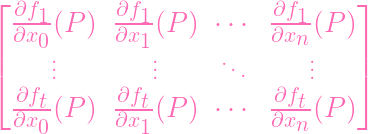(3) =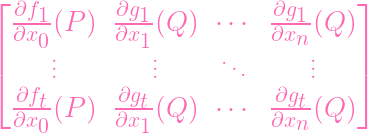(4) =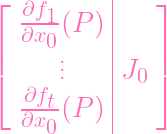(5)

So J0 is a submatrix of J. Hence

 rkJ ≥ rkJ0

Yaaaaaaaaay...... Wait that's only .... I need to show =.... Errrr.... LET'S GO BACK TO (1). And actually, I'm gonna transpose it this time (which is fine).

 JT =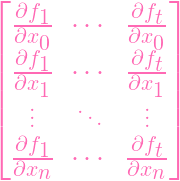Now, that top row is the pesky row that doesn't just "turn into J0" after we plug in P. However, I can use the hint and do something special with it. Using zero-based indexing, if I multiply the ith row by i and add every row to the first row, then Euler's lemma tells me I get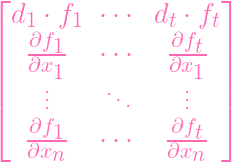And since fi(P) = 0 for all P, plugging in P turns this matrix into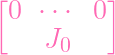(6)

Since J0 has rank n - r and can be put into an RREF with n - r pivots accordingly, and since the top row is a zero row, the RREF of this matrix has exactly n - r pivots. So the rank is EQUAL (=) to n - r

### ⇐=

So this time we're assuming that rkJ = n - r. Again assuming out point is in U0, we can reduce to the affine Y 0 given by the gis, and showing that P is nonsingular in Y 0 is equivalent to proving that rkJ0 = n - r. So now our goal is showing rkJ = n - rrkJ0 = n - r. Using the same logic, we can row-reduce J to (6). This time we know that the whole matrix has rank n - r. But since the top row is just 0, we know the RREF of the rest of the matrix has n-r pivots. I.e. rkJ0 = n-r. PWNED (actually no, that wasn't slick at all; I shouldn't have had to even split this into its component implications).

Hmmm? Oh, I put off showing that rank is independent of homogenous coordinates. Heh. Well, now the time has come...... TO RUN AWAY.